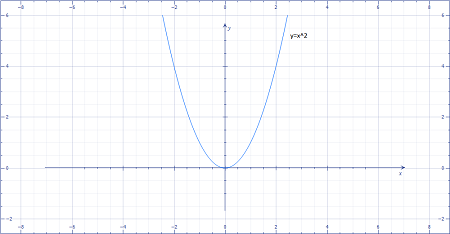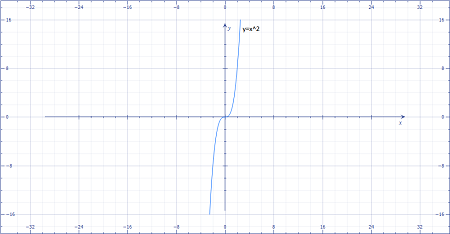# Let f(x) = x^2 and g(x) = x^3. Use these graphs to sketch the graph of the following functions....

## Question:

Let {eq}f(x) = x^2 {/eq} and {eq}g(x) = x^3 {/eq}. Use these graphs to sketch the graph of the following functions.

A) {eq}h(x) = -(x - 1)^2 + 2 {/eq}

B) {eq}p(x) = (3 - x)^3 - 4 {/eq}

## Graph of the Function:

If we have been given a function f(x) then -f(x) gives the reflection of the graph along the line y=0, that is, reflected toward y-axis and f(-x) gives the reflection of the graph along the line x=0, that is reflected toward x-axis.

Given graphs,

{eq}f(x) = x^2\\ g(x) = x^3 {/eq}

The graph of f(x) and g(x) is given as:andPart A

{eq}h(x) = -(x - 1)^2 + 2\\ \text{We...

Become a Study.com member to unlock this answer! Create your account